### Nano Res.│巨新/万初斌团队： “内在机理决定性能表现”，超薄多孔Co₃V₂O₈纳米片用于高能量密度水系钠离子电容器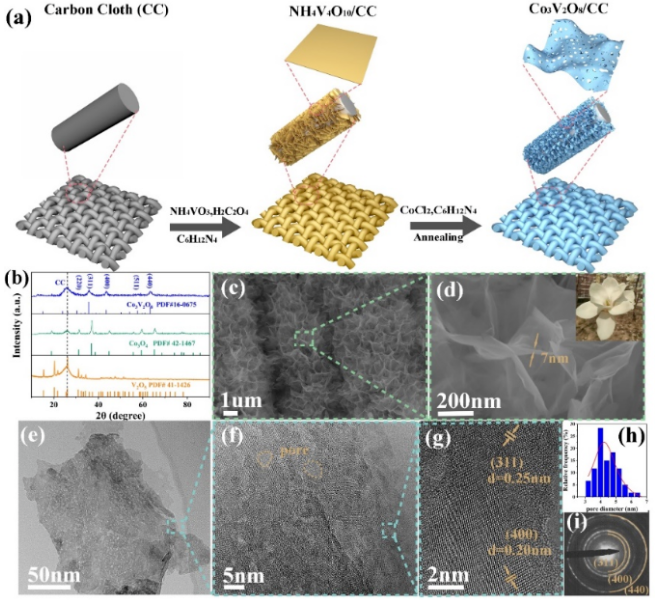Figure 1 (a) Schematic of fabricating the Co3V2O8 nanosheet. (b) XRD pattern of V2O5, Co3O4, and Co3V2O8. (c) and (d) SEM, (e) TEM, (f) and (g) HRTEM, (h) pore size distribution, and (i) SAED pattern of Co3V2O8.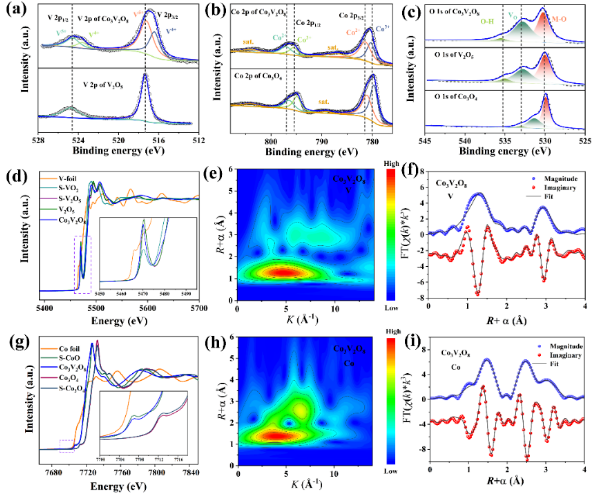Figure 2 High-resolution XPS spectra of (a) V2p of V2O5 and Co3V2O8. (b) Co 2p of Co3O4 and Co3V2O8. (c) O1s. (d) V k-edge EXENS for Co3V2O8, V2O5, and the guide sample. (e) Wavelet transform of V in Co3V2O8. (f) V k-edge EXAFS (points) and curve fit (line) for Co3V2O8 shown in R-space (FT magnitude and imaginary component). The data are k3-weighted and not phase corrected. (g) Co k-edge EXENS for Co3V2O8, Co3O4, and the guide sample. (h) Wavelet transform of Co in Co3V2O8. (i) Co k3-weighted R-space EXAFS and curve fit for Co3V2O8.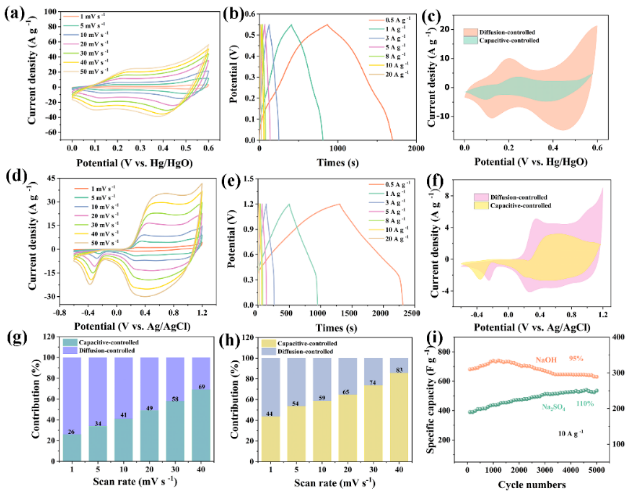Figure 3 Electrochemical properties of binder-free Co3V2O8 measured in 1 M NaOH and Na2SO4 electrolyte with a three-electrode system. (a) and (b) CV and GCD curves tested in NaOH at different scan rates and current densities of 1–50 mV s−1 and 0.5–20 A g−1, respectively. (c) Capacitive contributions in NaOH at a scan rate of 5 mV s−1. (d) and (e) CV and GCD curves in Na2SO4. (f) Capacitive contributions in Na2SO4 at a scan rate of 5 mV s−1. (g) and (h) Contribution ratio of capacitive- and diffusion-limited capacities in NaOH and Na2SO4 at different scan rates. (i) Cycling performance of Co3V2O8 in NaOH and Na2SO4 after 5000 cycles.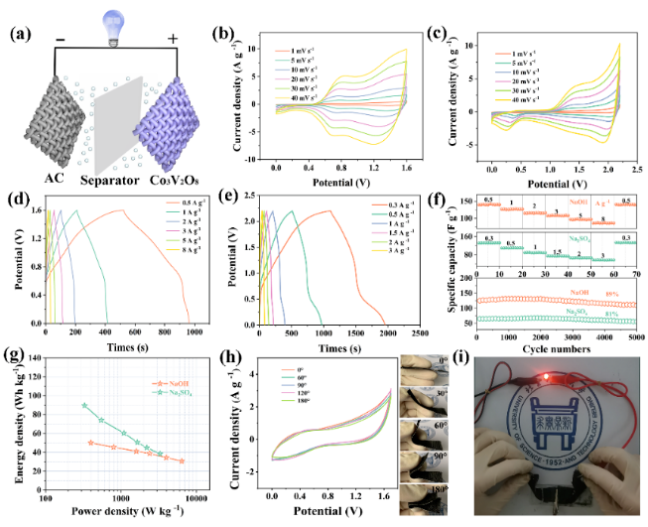Figure 4 (a) Schematic of a hybrid capacitor. (b) and (c) CV curves of Co3V2O8 in NaOH and Na2SO4 at different scan rates. (d) and (e) GCD curves of Co3V2O8 in NaOH and Na2SO4. (f) Rate capability and cycling stability and (g) Ragone plot of Co3V2O8 in NaOH and Na2SO4. (h) CV curves at 10 mV s-1 collected at different bending states. (i) Photograph of a LED indicator powered by the hybrid capacitors.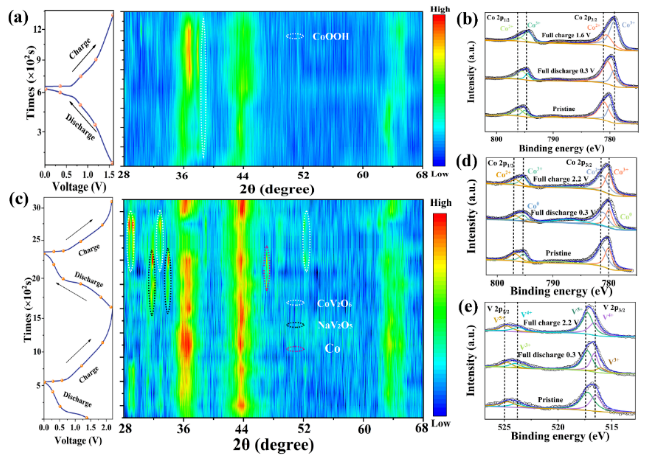Figure 5 (a) XRD spectra and corresponding GCD curves at charging and discharging states in the NaOH electrolyte. (b) Co 2p XPS spectra of Co3V2O8 in the NaOH electrolyte at charge/discharge states. (c) XRD spectra and corresponding GCD curves at charging and discharging states in Na2SO4 solution. (d) V 2p and (e) Co 2p XPS spectra of Co3V2O8 at charge/discharge states in Na2SO4 solution.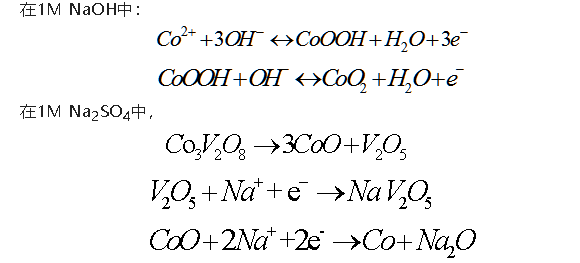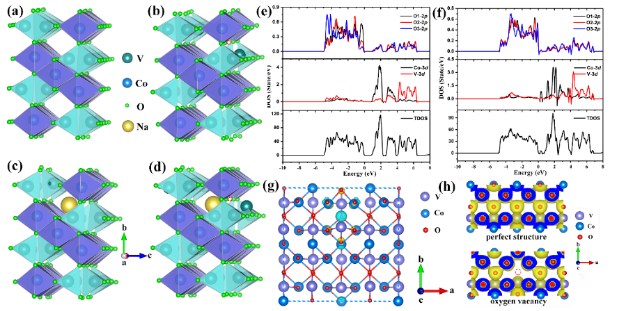Figure 6. Structure models of (a) perfect Co3V2O8, (b) Co3V2O8-Ov (Ov represents oxygen vacancy [red circle]), (c) Na-inserted Co3V2O8, and (d) Na-inserted Co3V2O8-Ov. Calculated TDOS and PDOS of (e) Co3V2O8 and (f) Co3V2O8-Ov. (g) Charge density difference isosurface plot of Co3V2O8 and Co3V2O8-Ov; the yellow and cyan colors correspond to charge accumulation and charge depletion, respectively. (h) Charge density plots of Co3V2O8 and Co3V2O8-Ov structures, respectively.

Anchun Tang, Chubin Wan*, Xianhe Meng, Xiangcao Li, Xiaoyu Hu, Miaofeng Huang & Xin Ju*Oxygen vacancies confined in porous Co3V2O8 sheets for durable and high-energy aqueous sodium-ion capacitors. Nano Research https://doi.org/10.1007/s12274-022-4147-3.down
wechat